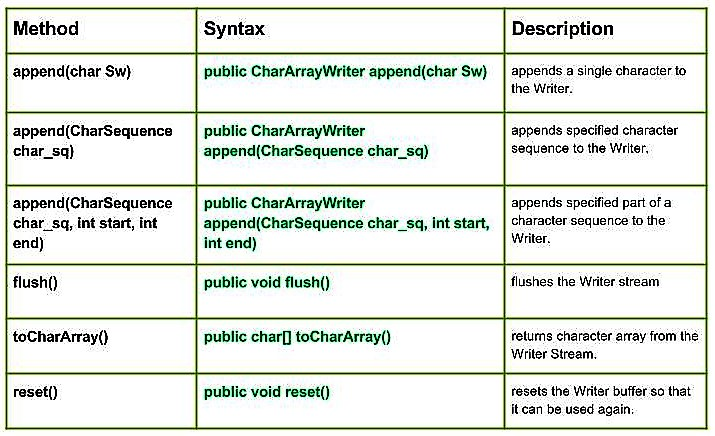Open in App
Not now

# Java.io.CharArrayWriter class in Java | Set 2

• Last Updated : 17 Jan, 2017

CharArrayWriter class in Java | Set 1Methods:

• append (char Sw) : java.io.CharArrayWriter.append(char Sw) appends a single character to the Writer.
Syntax:

```public CharArrayWriter append(char Sw)
Parameters :
Sw : character to be append
Return  :
CharArrayWriter```
• append (CharSequence char_sq) : java.io.CharArrayWriter.append(CharSequence char_sq) appends specified character sequence to the Writer.
Syntax:

```public CharArrayWriter append(CharSequence char_sq)
Parameters :
char_sq : Character sequence to append.
Return  :
CharArrayWriter, if char sequence is null, then NULL appends to the Writer. ```
• append (CharSequence char_sq, int start, int end) : java.io.CharArrayWriter.append(CharSequence char_sq, int start, int end) appends specified part of a character sequence to the Writer.
Syntax:

```public CharArrayWriter append(CharSequence char_sq, int start, int end)
Parameters :
char_sq : Character sequence to append.
start : start of character in the Char Sequence
end : end of character in the Char Sequence
Return  :
void```
• flush() : java.io.CharArrayWriter.flush() flushes the Writer stream
Syntax:

```public void flush()
Parameters :
-----
Return  :
void```
• toCharArray() : java.io.CharArrayWriter.toCharArray() returns character array from the Writer Stream.
Syntax:

```public char[] toCharArray()
Parameters :
-----
Return  :
void```
• reset() : java.io.CharArrayWriter.reset() resets the Writer buffer so that it can be used again.
Syntax:

```public void reset()
Parameters :
-----
Return  :
void```
• Java program illustrating use of CharArrayWriter class methods :

 `// Java program illustrating the working of CharArrayWriter class methods``// append(CharSequence char_sq), append(char Sw)``// append(CharSequence char_sq, int start,int end)``// flush(), reset(), toCharArray`` ` `import` `java.io.*;`` ` `public` `class` `NewClass``{``    ``public` `static` `void` `main(String[] args) ``throws` `IOException``    ``{``        ``// Initializing String Witer``        ``CharArrayWriter geek_writer1 = ``new` `CharArrayWriter();``        ``CharArrayWriter geek_writer2 = ``new` `CharArrayWriter();``        ``CharArrayWriter geek_writer3 = ``new` `CharArrayWriter();`` ` `        ``char``[] Sw = {``'G'``,``'E'``,``'E'``,``'K'``,``'S'``};`` ` `        ``for``(``char` `c: Sw)``        ``{``            ``// Use of append(char Sw) :``            ``geek_writer1 .append(c);`` ` `            ``System.out.println(``"append(char Sw) :"` `+ geek_writer1 .toString());``        ``}`` ` `        ``// Using flush() method``        ``geek_writer1.flush();``        ``System.out.println(``"\nUsing flush() : "``+ geek_writer1.toString());``        ``System.out.println(``""``);`` ` ` ` `        ``// Initializing Character Sequence``        ``CharSequence char_sq1 = ``"1 Hello 1"``;``        ``CharSequence char_sq2 = ``" : 2 Geeks 2"``;`` ` `        ``// Use of append(CharSequence char_sq)``        ``geek_writer2.append(char_sq1);``        ``geek_writer2.append(char_sq2);`` ` `        ``System.out.println(``"append(char_sq) : "` `+ geek_writer2.toString());`` ` `        ``// Use of append(CharSequence char_sq,int start,int end)``        ``geek_writer3.append(char_sq1, ``0``, ``3``);``        ``geek_writer3.append(char_sq2, ``3``, ``6``);`` ` `        ``System.out.println(``"append(char_sq,start,end) : "` `+ geek_writer3.toString());``        ``System.out.println(``""``);``         ` ` ` `        ``// Use of toCharArray() :``        ``char``[] toChar1 = geek_writer1.toCharArray();``        ``for` `(``char` `c1 : toChar1)``        ``{``            ``System.out.println(``"toCharArray : "``+ c1);``        ``}`` ` `        ``geek_writer1.reset();``        ``System.out.println(``"\nReset is invoked"``);``         ` ` ` `        ``char``[] toChar2 = geek_writer1.toCharArray();``        ``for` `(``char` `c2 : toChar2)``        ``{``            ``System.out.println(``"toCharArray : "``+ c2);``        ``}`` ` ` ` `        ``System.out.println(``"\ntoCharArray not working as reset is invoked"``);``    ``}``}`

Output :

```append(char Sw) :G
append(char Sw) :GE
append(char Sw) :GEE
append(char Sw) :GEEK
append(char Sw) :GEEKS

Using flush() : GEEKS

append(char_sq) : 1 Hello 1 : 2 Geeks 2
append(char_sq,start,end) : 1 H2 G

toCharArray : G
toCharArray : E
toCharArray : E
toCharArray : K
toCharArray : S

Reset is invoked

toCharArray not working as reset is invoked```

This article is contributed by Mohit Gupta 🙂. If you like GeeksforGeeks and would like to contribute, you can also write an article using contribute.geeksforgeeks.org or mail your article to contribute@geeksforgeeks.org. See your article appearing on the GeeksforGeeks main page and help other Geeks.